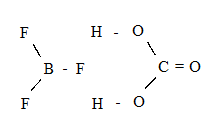# Among the following compounds the one that is polar and has the central atom with $s{p}^{2}$ hybridisation is

1. A

${H}_{2}C{O}_{3}$

2. B

$Si{F}_{4}$

3. C

$B{F}_{3}$

4. D

$HCl{O}_{2}$

FREE Lve Classes, PDFs, Solved Questions, PYQ's, Mock Tests, Practice Tests, and Test Series!

+91

Verify OTP Code (required)

I agree to the terms and conditions and privacy policy.

### Solution:

${H}_{2}C{O}_{3}$ and $B{F}_{3}$ both have triangular planar structure$B{F}_{3}$ is symmetrical

$\therefore \mu =0$ hence non polar, ${H}_{2}C{O}_{3}$is not symmetrical hence polar in nature.

## Related contentJoin Infinity Learn Regular Class Program!

Sign up & Get instant access to FREE PDF's, solved questions, Previous Year Papers, Quizzes and Puzzles!

+91

Verify OTP Code (required)

I agree to the terms and conditions and privacy policy.